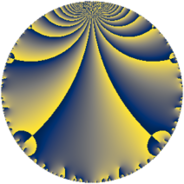# Properties

 Label 147.4.aLevel $147$ Weight $4$ Character orbit 147.a Rep. character $\chi_{147}(1,\cdot)$ Character field $\Q$ Dimension $20$ Newform subspaces $13$ Sturm bound $74$ Trace bound $5$

# Related objects

## Defining parameters

 Level: $$N$$ $$=$$ $$147 = 3 \cdot 7^{2}$$ Weight: $$k$$ $$=$$ $$4$$ Character orbit: $$[\chi]$$ $$=$$ 147.a (trivial) Character field: $$\Q$$ Newform subspaces: $$13$$ Sturm bound: $$74$$ Trace bound: $$5$$ Distinguishing $$T_p$$: $$2$$, $$5$$

## Dimensions

The following table gives the dimensions of various subspaces of $$M_{4}(\Gamma_0(147))$$.

Total New Old
Modular forms 64 20 44
Cusp forms 48 20 28
Eisenstein series 16 0 16

The following table gives the dimensions of the cuspidal new subspaces with specified eigenvalues for the Atkin-Lehner operators and the Fricke involution.

$$3$$$$7$$FrickeDim.
$$+$$$$+$$$$+$$$$5$$
$$+$$$$-$$$$-$$$$5$$
$$-$$$$+$$$$-$$$$3$$
$$-$$$$-$$$$+$$$$7$$
Plus space$$+$$$$12$$
Minus space$$-$$$$8$$

## Trace form

 $$20 q + 4 q^{2} + 60 q^{4} + 16 q^{5} + 12 q^{6} + 48 q^{8} + 180 q^{9} + O(q^{10})$$ $$20 q + 4 q^{2} + 60 q^{4} + 16 q^{5} + 12 q^{6} + 48 q^{8} + 180 q^{9} + 28 q^{10} - 40 q^{11} - 24 q^{12} + 80 q^{13} - 84 q^{15} + 332 q^{16} - 120 q^{17} + 36 q^{18} - 40 q^{19} - 172 q^{20} - 132 q^{22} - 40 q^{23} + 324 q^{24} + 728 q^{25} - 172 q^{26} + 376 q^{29} + 288 q^{30} + 168 q^{31} + 168 q^{32} + 96 q^{33} - 276 q^{34} + 540 q^{36} - 508 q^{37} - 1360 q^{38} - 756 q^{39} + 924 q^{40} + 1016 q^{41} - 828 q^{43} - 1072 q^{44} + 144 q^{45} - 520 q^{46} - 552 q^{47} - 816 q^{48} - 292 q^{50} + 456 q^{51} + 1420 q^{52} - 784 q^{53} + 108 q^{54} - 952 q^{55} - 276 q^{57} - 124 q^{58} - 792 q^{59} - 204 q^{60} - 592 q^{61} + 1824 q^{62} + 156 q^{64} - 168 q^{65} + 1896 q^{66} + 148 q^{67} - 492 q^{68} - 144 q^{69} + 608 q^{71} + 432 q^{72} + 72 q^{73} + 1196 q^{74} + 624 q^{75} + 832 q^{76} - 1692 q^{78} + 1936 q^{79} - 3484 q^{80} + 1620 q^{81} - 1492 q^{82} - 2208 q^{83} + 3904 q^{85} - 9836 q^{86} + 1032 q^{87} - 5484 q^{88} - 920 q^{89} + 252 q^{90} - 2744 q^{92} + 1788 q^{93} + 1344 q^{94} - 416 q^{95} + 204 q^{96} + 744 q^{97} - 360 q^{99} + O(q^{100})$$

## Decomposition of $$S_{4}^{\mathrm{new}}(\Gamma_0(147))$$ into newform subspaces

Label Dim $A$ Field CM Traces A-L signs $q$-expansion
$a_{2}$ $a_{3}$ $a_{5}$ $a_{7}$ 3 7
147.4.a.a $1$ $8.673$ $$\Q$$ None $$-3$$ $$-3$$ $$3$$ $$0$$ $+$ $-$ $$q-3q^{2}-3q^{3}+q^{4}+3q^{5}+9q^{6}+\cdots$$
147.4.a.b $1$ $8.673$ $$\Q$$ None $$-3$$ $$3$$ $$-3$$ $$0$$ $-$ $+$ $$q-3q^{2}+3q^{3}+q^{4}-3q^{5}-9q^{6}+\cdots$$
147.4.a.c $1$ $8.673$ $$\Q$$ None $$-3$$ $$3$$ $$18$$ $$0$$ $-$ $-$ $$q-3q^{2}+3q^{3}+q^{4}+18q^{5}-9q^{6}+\cdots$$
147.4.a.d $1$ $8.673$ $$\Q$$ None $$-1$$ $$-3$$ $$12$$ $$0$$ $+$ $-$ $$q-q^{2}-3q^{3}-7q^{4}+12q^{5}+3q^{6}+\cdots$$
147.4.a.e $1$ $8.673$ $$\Q$$ None $$-1$$ $$3$$ $$-12$$ $$0$$ $-$ $-$ $$q-q^{2}+3q^{3}-7q^{4}-12q^{5}-3q^{6}+\cdots$$
147.4.a.f $1$ $8.673$ $$\Q$$ None $$4$$ $$-3$$ $$-18$$ $$0$$ $+$ $-$ $$q+4q^{2}-3q^{3}+8q^{4}-18q^{5}-12q^{6}+\cdots$$
147.4.a.g $1$ $8.673$ $$\Q$$ None $$4$$ $$3$$ $$4$$ $$0$$ $-$ $-$ $$q+4q^{2}+3q^{3}+8q^{4}+4q^{5}+12q^{6}+\cdots$$
147.4.a.h $1$ $8.673$ $$\Q$$ None $$4$$ $$3$$ $$18$$ $$0$$ $-$ $-$ $$q+4q^{2}+3q^{3}+8q^{4}+18q^{5}+12q^{6}+\cdots$$
147.4.a.i $2$ $8.673$ $$\Q(\sqrt{57})$$ None $$-3$$ $$-6$$ $$-6$$ $$0$$ $+$ $-$ $$q+(-1-\beta )q^{2}-3q^{3}+(7+3\beta )q^{4}+\cdots$$
147.4.a.j $2$ $8.673$ $$\Q(\sqrt{2})$$ None $$2$$ $$-6$$ $$20$$ $$0$$ $+$ $+$ $$q+(1+\beta )q^{2}-3q^{3}+(-5+2\beta )q^{4}+\cdots$$
147.4.a.k $2$ $8.673$ $$\Q(\sqrt{2})$$ None $$2$$ $$6$$ $$-20$$ $$0$$ $-$ $+$ $$q+(1+\beta )q^{2}+3q^{3}+(-5+2\beta )q^{4}+\cdots$$
147.4.a.l $3$ $8.673$ 3.3.57516.1 None $$1$$ $$-9$$ $$11$$ $$0$$ $+$ $+$ $$q+\beta _{1}q^{2}-3q^{3}+(8+\beta _{1}+\beta _{2})q^{4}+\cdots$$
147.4.a.m $3$ $8.673$ 3.3.57516.1 None $$1$$ $$9$$ $$-11$$ $$0$$ $-$ $-$ $$q+\beta _{1}q^{2}+3q^{3}+(8+\beta _{1}+\beta _{2})q^{4}+\cdots$$

## Decomposition of $$S_{4}^{\mathrm{old}}(\Gamma_0(147))$$ into lower level spaces

$$S_{4}^{\mathrm{old}}(\Gamma_0(147)) \cong$$ $$S_{4}^{\mathrm{new}}(\Gamma_0(7))$$$$^{\oplus 4}$$$$\oplus$$$$S_{4}^{\mathrm{new}}(\Gamma_0(21))$$$$^{\oplus 2}$$$$\oplus$$$$S_{4}^{\mathrm{new}}(\Gamma_0(49))$$$$^{\oplus 2}$$